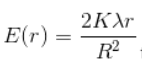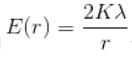# Problem: A very long solid cylinder of radius R has a positive charge uniformly distributed throughout it, with a charge per unit volume λ. From the expression for E for this cylinder: for r≤R and for r≥RPart A. Derive the expression for the electric field INSIDE the volume at a distance r≥R from the axis of the cylinder in terms of the charge density ρ. Express your answer in terms of the given quantities and appropriate constants.Part B. What is the electric field at a point OUTSIDE the volume in terms of the charge per unit length λ in the cylinder? Express your answer in terms of the given quantities and appropriate constants.Let V = 0 at the surface of the cylinder. In each case, express your result in terms of the charge per unit length λ of the charge distribution.

###### FREE Expert Solution

From Gauss law, the net electric field that comes out of an enclosed surface is directly proportional to the charge enclosed inside the surface.

88% (257 ratings)###### Problem Details

A very long solid cylinder of radius R has a positive charge uniformly distributed throughout it, with a charge per unit volume λ. From the expression for E for this cylinder:for r≤R andfor r≥R

Part A. Derive the expression for the electric field INSIDE the volume at a distance r≥R from the axis of the cylinder in terms of the charge density ρ. Express your answer in terms of the given quantities and appropriate constants.
Part B. What is the electric field at a point OUTSIDE the volume in terms of the charge per unit length λ in the cylinder? Express your answer in terms of the given quantities and appropriate constants.

Let V = 0 at the surface of the cylinder. In each case, express your result in terms of the charge per unit length λ of the charge distribution.

Frequently Asked Questions

What scientific concept do you need to know in order to solve this problem?

Our tutors have indicated that to solve this problem you will need to apply the Gauss' Law concept. You can view video lessons to learn Gauss' Law. Or if you need more Gauss' Law practice, you can also practice Gauss' Law practice problems.